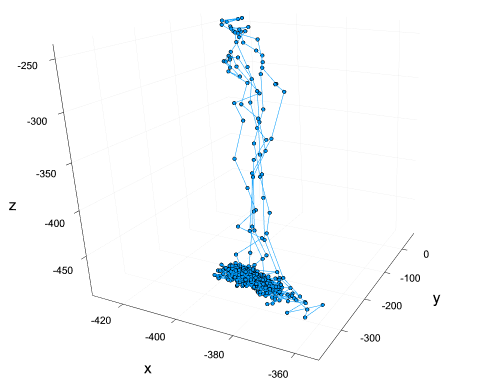# How to plot 3d data while using a gradient color depending on the "time" of the point?

Sorry for the confusing title, I’m not really sure how to summarize it. Basically, I have a list of 3D points which are order according the time these points were taken. In a sense, you can think of these as 4D points, where one of the coordinates is time. It looks something like this:I would like for the time coordinate to be inferred using a gradient color of each point. I’m using `Plots.jl` and the only way I’ve found to do this is by passing a vector to the attribute `marker_z`. The problem is that I have 600 points, thus the vector passed to `marker_z` is of length 600: this completely breaks the `plotlyjs()` backend I’m using (which is useful because it lets me interact and rotate the 3D plot): the plot doesn’t load at all. It works for a handful of points, but in my case it is not viable.
Is there a solution to this problem? Some other package or backend that lets me interact with the plot and have the points color respect the time series?

I would say that GLMakie is your friend on this one !
It can handle large amounts of data, while being interactive

600 points is not that much, PlotlyJS should handle that easily. Maybe try to use it directly, without the Plots.jl package. Here’s the documentation for the 3d plot.